A meter pole perpendicular to the ground throws a shadow of 40 cm long, the house throws a shadow 6 meters long. What is the height of the house?

h2 =  15 m

### Step-by-step explanation: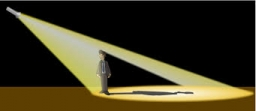Did you find an error or inaccuracy? Feel free to write us. Thank you!Tips to related online calculators
Check out our ratio calculator.

#### You need to know the following knowledge to solve this word math problem:

We encourage you to watch this tutorial video on this math problem:

## Related math problems and questions:

• The chimney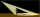The chimney casts a shadow 45 meters long. The one-meter-long rod standing perpendicular to the ground has a shadow 90 cm long. Calculate the height of the chimney.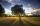Nine meters height poplar tree has a shadow 16.2 meters long. How long shadow have at the same time Joe if he is 1,4m tall?Tree perpendicular to the horizontal surface has a shadow 8.32 meters long. At the same time meter rod perpendicular to the horizontal surface has shadow 64 cm long. How tall is tree?The shadow of the tree is 16 meters long. Shadow of two meters high tourist sign beside standing is 3.2 meters long. What height has tree (in meters)?
• Sun raysIf the sun's rays are at an angle 60° then famous Great Pyramid of Egypt (which is now high 137.3 meters) has 79.3 m long shadow. Calculate current height of neighboring chefren pyramid whose shadow is measured at the same time 78.8 m and the current heig
• Vertical rodThe vertical one-meter-long rod casts a shadow 150 cm long. Calculate the height of a column whose shadow is 36 m long at the same time.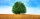The shadow of a 1 m high pole thrown on a horizontal plane is 0.8 m long. At the same time, the shadow of a tree thrown on a horizontal plane is 6.4 m. Determine the height of the tree.Miro stands under a tree and watching its shadow and shadow of the tree. Miro is 180 cm tall and its shade is 1.5 m long. The shadow of the tree is three times as long as Miro's shadow. How tall is the tree in meters?The nine-meter poplar casts a shadow 16.2 m long. How long does a shadow cast by Peter at the same time, if it is 1.4 m high?
• Sides od triangleSides of the triangle ABC has length 4 cm, 5 cm and 7 cm. Construct triangle A'B'C' that are similar to triangle ABC which has a circumference of 12 cm.
• Trapezium diagonalsIt is given trapezium ABCD with bases | AB | = 12 cm, |CD| = 8 cm. Point S is the intersection of the diagonals for which |AS| is 6 cm long. Calculate the length of the full diagonal AC.
• Rectangular trianglesThe lengths of corresponding sides of two rectangular triangles are in the ratio 2:5. At what ratio are medians relevant to hypotenuse these right triangles? At what ratio are the contents of these triangles? Smaller rectangular triangle has legs 6 and 8
• Similarity coefficientIn the triangle TMA the length of the sides is t = 5cm, m = 3.5cm, a = 6.2cm. Another similar triangle has side lengths of 6.65 cm, 11.78 cm, 9.5 cm. Determine the similarity coefficient of these triangles and assign similar sides to each other.
• SimilarityABC is a triangle wherein a = 4 cm, b = 6 cm, c = 8 cm. Is it similar to the triangle DEF: d = 3 cm, e = 4.5 cm, f = 6 cm? If so, determine the ratio of similarity.
• MastMast has 13 m long shadow on a slope rising from the mast foot in the direction of the shadow angle at angle 13.3°. Determine the height of the mast, if the sun above the horizon is at angle 45°12'.
• Chimney and treeCalculate the height of the factory chimney, which casts a shadow 6.5 m long in the afternoon. At the same time, a 6 m high tree standing near it casts a shadow 25 dm long.
• Column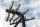Perpendicular pole high 8 m tall broke and its toe fell 2.7 m from the bottom of the pole. At what height above the ground pole broke?